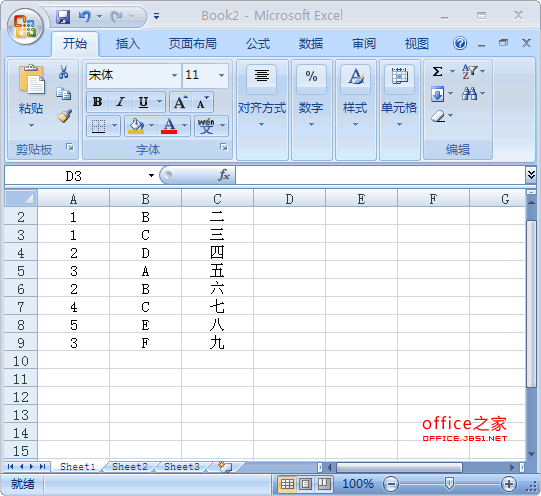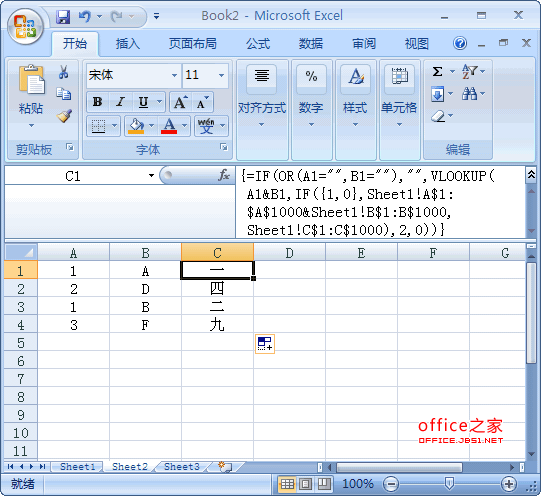# Excel中多条件查找对满足两个以上条件的数据进行查找并引用SHEET2工作表C1单元格使用以下数组公式，可达到目的：

=IF(OR(A1="",B1=""),"",OFFSET(Sheet1!\$C\$1,SUM(IF((Sheet1!A\$1:A\$1000=A1)*(Sheet1!B\$1:B\$1000=B1),ROW(Sheet1!C\$1:C\$1000),0))-1,0,1,1))

=IF(OR(A1="",B1=""),"",VLOOKUP(A1&B1,IF({1,0},Sheet1!A\$1:\$A\$1000&Sheet1!B\$1:B\$1000,Sheet1!C\$1:C\$1000),2,0))

=IF(OR(A1="",B1=""),"",INDEX(Sheet1!C\$1:C\$1000,MATCH(A1&B1,Sheet1!A\$1:A\$1000&Sheet1!B\$1:B\$1000,0)))

=IF(OR(A1="",B1=""),"",INDIRECT("Sheet1!\$C"&SUMPRODUCT((Sheet1!A\$1:A\$1000=A1)*(Sheet1!B\$1:B\$1000=B1)*ROW(Sheet1!C\$1:C\$1000))))

=IF(OR(A1="",B1=""),"",LOOKUP(2,1/((Sheet1!A\$1:A\$1000=A1)*(Sheet1!B\$1:B\$1000=B1)),Sheet1!C\$1:C\$1000))

=IF(ISERROR(VLOOKUP(A1&B1,IF({1,0},Sheet1!A\$1:\$A\$1000&Sheet1!B\$1:B\$1000,Sheet1!C\$1:C\$1000),2,FALSE)),"",VLOOKUP(A1&B1,IF({1,0},Sheet1!A\$1:\$A\$1000&Sheet1!B\$1:B\$1000,Sheet1!C\$1:C\$1000),2,0))